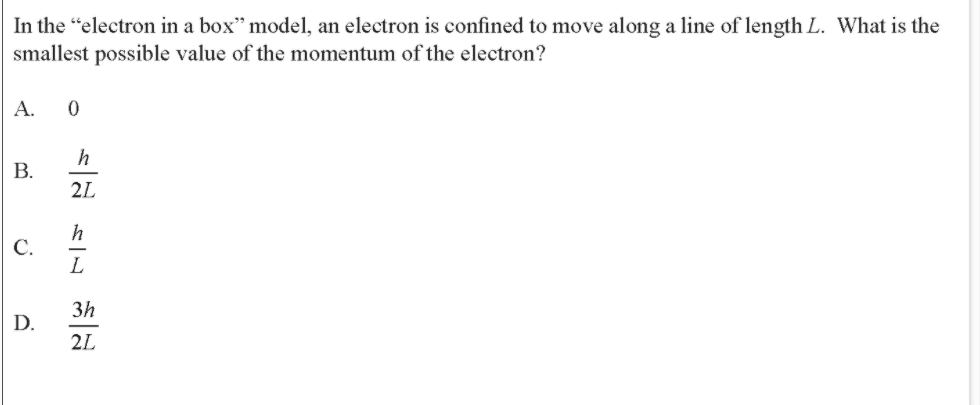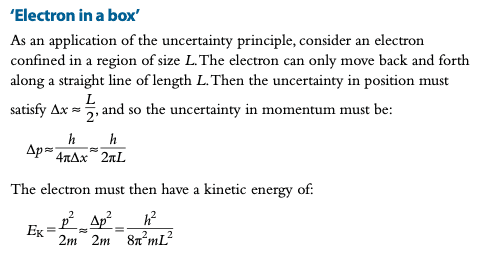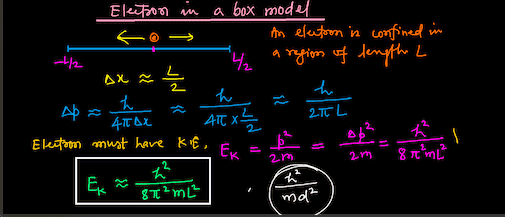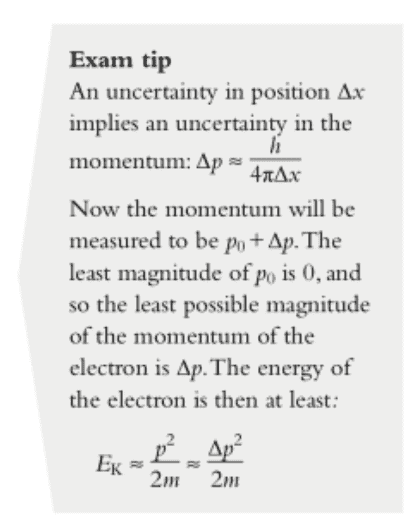# Momentum of Electron in a Box (IB Physics QM)

• lekh2003
In summary, In my physics textbooks, the solution to this problem is seen from a friend's teacher's work as well as from a standing wave method. However, very clearly, this is not an option. So then I considered the "standing wave" method, outline here:However, very clearly, this is not an option. So then I considered the "standing wave" method, outline here:With this method you get $$p = \frac{h}{2L}$$What is the correct method? What is happening? When do I use uncertainty principle and when do I treat the electron as a standing wave? Are they inconsistent?A measurement of momentum is effectively also a measurement of energy in

#### lekh2003

Gold Member
Homework Statement
Explained in full below
Relevant Equations
Explained in full belowHere's the question ^

My first thought to solving this is to use Heisenberg's uncertainty principle. $$\Delta x \Delta p = \frac{h}{4\pi}$$ Now, we approximate ##\Delta x = \frac{L}{2}##. Then, plug and chug we end up with:$$p =\frac{h}{2\pi L}$$
I thought this was it, especially because this solution is in my physics textbooksThis is also seen from a friend's teacher's work:However, very clearly, this is not an option. So then I considered the "standing wave" method, outline here: From 11:00 to 16:00

With this method you get $$p = \frac{h}{2L}$$

What is the correct method? What is happening? When do I use uncertainty principle and when do I treat the electron as a standing wave? Are they inconsistent?

Last edited:
Did you notice the ##\ \approx\ ## ?
What is the proper way to calculate <p> ?

Note that the exercise asks for p, not for ##\sqrt {p^2} ##

##\ ##

lekh2003 said:
What is the correct method? What is happening? When do I use uncertainty principle and when do I treat the electron as a standing wave? Are they inconsistent?
A measurement of momentum is effectively also a measurement of energy in this case. The correct method, I would say, is to consider the ground state of the system.

BvU said:
Note that the exercise asks for p

One might say that since p can be negative, the best answer is A, zero.

One might say that since p can be negative, the best answer is A, zero.
In that case you could say that zero is the only answer.

I can't say I necessarily understand what has been discussed in the thread so far.

The way I'm currently rationalising it is that the approximation of ##\Delta x = \frac L2## is causing the issues. Hence, we cannot use that approximation and the standing wave approach is more "accurate".

lekh2003 said:
However, very clearly, this is not an option

Why do you say this?

And why do you think the Heisenberg Uncertainty relation, which is an inequality, will give you an exact answer? Furthermore, it is an expression for the uncertainty on the momentum, not the momentum itself? Furthermore, since you can't tell the direction the particle is traveling, I don't see how the uncertainty on the momentum can be any smaller than twice the momentum.

•PeroK
lekh2003 said:
I can't say I necessarily understand what has been discussed in the thread so far.
We helpers have a problem with the formulation of the exercise: it is unclear if the book asks for an average momentum (which would be 0 from symmetry alone) or some |p| , so yes, this might be confusing for you.

The way I'm currently rationalising it is that the approximation of ##\Delta x = \frac L2## is causing the issues. Hence, we cannot use that approximation and the standing wave approach is more "accurate".
##\ \Delta x = \frac L2\ ## is a coarse approximation. The wave function has a shape that makes it smaller.

Proper way to calculate ##\ \Delta x \ ## https://www.colby.edu/chemistry/PChem/notes/PrtinboxHeisenbergn.pdf; result ##\displaystyle{\sigma_x = \frac{L}{2\pi} \sqrt{\frac{\pi^2}{3}-2\ }}## for n = 1.

Same link: ##\displaystyle{\sigma_p = \frac{h}{2L}}\ ## for n = 1. Matches your standing wave in #1.

Result for ##\ \sigma_p \sigma_x \ ## is ##\displaystyle {1.136 \;{\hbar\over 2} }##

Proper way to calculate <p> here

Look at it from the bright side: if you work through all that, you really have learned quite a bit!

##\ ##

•PeroK
lekh2003 said:
I can't say I necessarily understand what has been discussed in the thread so far.

The way I'm currently rationalising it is that the approximation of ##\Delta x = \frac L2## is causing the issues. Hence, we cannot use that approximation and the standing wave approach is more "accurate".
It's not a question of accuracy. Every system has a ground state, which has the lowest energy. In the case of the particle in a box the lowest energy is the same as the lowest magnitude of momentum.

QM is a lot more than the Heisenberg Uncertainty Principle.

Guys the question doesn't ask for ##\Delta p## neither for ##<p>## it asks for the smallest possible value of ##p##.

Delta2 said:
Guys the question doesn't ask for ##\Delta p## neither for ##<p>## it asks for the smallest possible value of ##p##.
And what is your interpretation ?
Note that there are no negative numbers among the choices

BvU said:
And what is your interpretation ?
Note that there are no negative numbers among the choices
My interpretation is that it asks for the absolute value of the smallest possible value...•rsk
so ##\sqrt {p^2}\; ## ?

edit

or ##\displaystyle {\Biggl | \min \Bigl ( - i\hbar {\partial \over \partial x} \psi \Bigr ) \Biggr | }\ ## as you say ?

or ##\displaystyle {\min \Biggl | \Bigl ( - i\hbar {\partial \over \partial x} \psi \Bigr ) \Biggr | }\ ## ?

•Delta2
lekh2003 said:
Homework Statement:: Explained in full below
Relevant Equations:: Explained in full below

View attachment 279475
Here's the question ^

My first thought to solving this is to use Heisenberg's uncertainty principle. $$\Delta x \Delta p = \frac{h}{4\pi}$$ Now, we approximate ##\Delta x = \frac{L}{2}##. Then, plug and chug we end up with:$$p =\frac{h}{2\pi L}$$
I thought this was it, especially because this solution is in my physics textbooks
View attachment 279476
This is also seen from a friend's teacher's work:
View attachment 279478

However, very clearly, this is not an option. So then I considered the "standing wave" method, outline here: From 11:00 to 16:00

With this method you get $$p = \frac{h}{2L}$$

What is the correct method? What is happening? When do I use uncertainty principle and when do I treat the electron as a standing wave? Are they inconsistent?
Definitely the standing wave method is the one to go with here and B is definitely the correct answer. If you watch the video a little beyond the 16 min mark, he actually looks at a question very similar to this one.

As @Delta2 points out, it's asking for smallest value of p, not Δp.

If you look back through IB past multiple choice papers, this Q appears fairly often so there are plenty of other examples you can use to check.

•Delta2
Why do you say this?
I meant that it isn't an option in the set of answers for the multiple choice. I don't see any fundamental issue.

rsk said:
As @Delta2 points out, it's asking for smallest value of p, not Δp.
Normally, I would agree, and throughout my study of this topic, I have followed this. But then one fine day I read the textbook and find a question where they approximate it directly. For that reason, I considered the alternate method. And the textbook also used that so ¯\_(ツ)_/¯rsk said:
If you look back through IB past multiple choice papers, this Q appears fairly often so there are plenty of other examples you can use to check.
I do know this, but it was still confusing.

After all these posts I seem to still be confused. What I understand is that the method using the uncertainty principle is finding some momentum lower bound based on uncertainties, but the standing wave method is finding an actual momentum lower bound based on wave formations?

Which is correct? I still don't understand what is happening, I'd appreciate if one person could summarise and explain.

I also fully understand that the question is poorly defined, and that happens to be the case for most of the questions and topics in this syllabus, which annoys me significantly. I understand if the technical conclusion is that the question isn't well defined or some complex calculus related answer. But within the confines of my syllabus (which for some reason doesn't have ANY calculus), what is the explanation?

•Delta2
lekh2003 said:
View attachment 279630

Which is correct? I still don't understand what is happening, I'd appreciate if one person could summarise and explain.
To summarise:

1) This is Quantum Mechanics; and the correct approach is the fully quantum mechanical one: system - ground state - momentum.

2) The above exam tip is, at best, a shortcut that takes advantage of a numerical coincidence in such a simple system. And, at worst, an abuse of the principles of QM. Thinking like that in QM is a dead end, as it cannot be extended to systems generally.

Note that all the uncertainty in the momentum is directional. E.g. if we take the ground state energy of $$E_1 = \frac{h^2}{8mL^2}$$ then we have two possible, equally likely, values of momentum: $$p = \pm\frac{h}{2L}$$ Note that there is no uncertainty in the magnitude of momentum, only in the direction of the momentum.

Now, to compute the uncertainty in ##p## we have: $$\langle p \rangle = 0, \ \ \text{and} \ \ \langle p^2 \rangle = \frac{h^2}{4L^2}$$ Hence: $$\sigma^2_p = \langle p^2 \rangle - \langle p \rangle^2 = \frac{h^2}{4L^2}$$ And $$\sigma_p = \frac{h}{2L}$$

•lekh2003, BvU and Delta2
PeroK said:
Note that all the uncertainty in the momentum is directional. E.g. if we take the ground state energy of $$E_1 = \frac{h^2}{8mL^2}$$ then we have two possible, equally likely, values of momentum: $$p = \pm\frac{h}{2L}$$

Wondered if you could explain this part to me. Doesn't a Fourier transform of wavefunction of particle in a box in position rep gives wavefunction in momentum rep which has a continuous spectrum? In other words I thought the momentum in the energy eigenstate with eigenvalue ##E_n## isn't constrained to be ##\pm \hbar k_n##.

etotheipi said:
Wondered if you could explain this part to me. Doesn't a Fourier transform of wavefunction of particle in a box in position rep gives wavefunction in momentum rep which has a continuous spectrum? In other words I thought the momentum in the energy eigenstate with eigenvalue ##E_n## isn't constrained to be ##\pm \hbar k_n##.
I haven't ever looked at that. Perhaps it's related to the problem that the momentum operator is not well-defined on the Hilbert space here?

I think the factor ##k_n = n\pi / L##, the wavenumber of the position wavefunction which is related to the energy eigenvalues, cannot here be related to the quantity ##p / \hbar## - unlike in the case for a free particle.

and one reason is that the momentum is not discretised. or in other words, to construct the sinusoidal position wave-function confined to the region ##-L/2 < x < L/2## only, you need to add together an infinite number of waves, and heuristically when you take the Fourier transform you end up with a continuous spectrum.

there might be a deeper reason, but I don't know nearly enough about this to commentI think it is safe to consider two possibilities here: (a) declare this question "bad" in the sense that no correct answer can be found among the four or at all or (b) accept it as "good" in which case we know that only one of the four eigenanswers has an outcome probability of 1 when the measurement (attempt to answer the question) is made.

It seems to me that there is an uncertainty principle of sorts at work here: the more homework helpers attempt to answer this multiple choice question, the more uncertain what is being asked becomes. That leads me to believe that none of the four choices is an eigenanswer, let alone having a probability of 1. It is, therefore, my opinion that this question is "bad".

•BvUWhat happened ?

etotheipi said:
Wondered if you could explain this part to me. Doesn't a Fourier transform of wavefunction of particle in a box in position rep gives wavefunction in momentum rep which has a continuous spectrum? In other words I thought the momentum in the energy eigenstate with eigenvalue ##E_n## isn't constrained to be ##\pm \hbar k_n##.
I find this a good and interesting question. IIRC the spectrum (i.e. the probability distribution of possible outcomes of measurements) consists of eigenvalues of the momentum operator, with eigenfunctions that satisfy $$-i\hbar {\partial \phi \over \partial x} = p\,\phi$$where ##\ p\ ## is the eigenvalue. Such ##\phi## are ##\ \displaystyle {{1\over L}e^{ipx/\hbar}}\ ## so I think we need $$\int_0^L {{1\over\sqrt L}e^{ipx/\hbar}} \, \sqrt{2\over L}\sin \left ({n\pi\over L} x\right ) \;dx$$ so functions of ##p## to get the p-spectrum ?

##\ ##

lekh2003 said:
After all these posts I seem to still be confused. What I understand is that the method using the uncertainty principle is finding some momentum lower bound based on uncertainties, but the standing wave method is finding an actual momentum lower bound based on wave formations?

Which is correct? I still don't understand what is happening, I'd appreciate if one person could summarise and explain.
You've got it essentially correct. It turns out this problem is a bit more complicated when you look into it more deeply (posts 17, 18, and 19), which can cast some doubt on the stuff said in earlier posts, but you can ignore that for now.

There are technical definitions of ##\Delta x## and ##\Delta p##, and if you calculate them for a given state, you'll find they satisfy the uncertainty principle. (Post #8)

But as you did, you can also estimate ##\Delta x## based on physical grounds, and then use the uncertainty principle to obtain an estimate for ##\Delta p##. For the particle in a box, you also know the average momentum is 0, so ##(\Delta p)^2## gives you an estimate of ##p^2## and therefore ##\lvert p \rvert##. (Post #16 in reverse.) But your results here are going to be estimates, so you shouldn't be surprised that using it didn't yield one of the answer choices. (Post #7) Moreover, this line of reasoning didn't consider the state of the particle at all, so that's another clue it will yield at best an estimate rather than an exact value.

Looking at the wave function, one might attribute a wavelength of ##\lambda = 2L## to the ground state. Using the deBroglie relation, you then get ##\lvert p \rvert = h/\lambda = h/2L##. Or if you look at the ground-state energy and set that to ##p^2/2m##, you find the same result. This was the intent of the question, which should have more correctly referred to the minimum magnitude of the momentum (but that bit of sloppiness in language is fairly common).

•lekh2003 and BvU